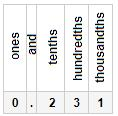Courses

Olympiad Test: Decimals And Fractions - 2

20 Questions MCQ Test Math Olympiad for Class 5 | Olympiad Test: Decimals And Fractions - 2

Description
Attempt Olympiad Test: Decimals And Fractions - 2 | 20 questions in 40 minutes | Mock test for Class 5 preparation | Free important questions MCQ to study Math Olympiad for Class 5 for Class 5 Exam | Download free PDF with solutions
QUESTION: 1

Which of the following is equal to 9,842.1039?

Solution:

Explanation:- 9,842.1039 :- Nine thousand, eight hundred, forty-two and one thousand, thirty nine ten-thousandths.

QUESTION: 2

Solution:
QUESTION: 3

Which of the following is the smallest decimal number?

Solution:
QUESTION: 4

What is the hundredths digit in the number 356.812?

Solution:

The hundredths digit is the second digit to the right of the decimal point, which is the 1: 356.812

QUESTION: 5

For the decimal number 83.72 what is the digit in the hundredths place?

Solution:

The tenths place comes immediately after the decimal point and the hundredths place comes after that. In this case the digit in the tenths place is 7 and the digit in the hundredths place is 2

QUESTION: 6

Which of the following is the largest decimal number?

Solution:

231/1000 is shown in place value chart below.QUESTION: 7

Which of the following choices lists these decimals in order from least to greatest: 0.910, 0.091, 0.9?

Solution:
QUESTION: 8

Which of the following choices lists these decimals in order from least to greatest : 3.45, 3.0459, 3.5, 3.4059?

Solution:
QUESTION: 9

(0.1 + 0.01) (0.1 − 0.01) =

Solution:
QUESTION: 10

For the number 489.6327, where do you find the smallest digit?

Solution:

The number in the hundredths place is the second digit after the decimal point. Only 87.349 has a 4 in the hundredths place

QUESTION: 11

Which of the following choices lists these decimals in order from least to greatest :  7.102, 7.0102, 7.012, 7.00102, 7.102021?

Solution:
QUESTION: 12

What are the number 2.0847 to 2 decimal places?

Solution:
QUESTION: 13

For the number 36.2495, what is the place value of the digit 9?

Solution:

9 is the third digit to the right of the decimal point, so it is 9 thousandths.(2 is in the tenths place, 4 is in the hundredths place, 9 is in the thousandths place and 5 is in the ten-thousandths place)

QUESTION: 14

What is the tenths digit in the number 43.765?

Solution:

The tenths digit is the first digit to the right of the decimal point, which is the 7.

QUESTION: 15

Solution:
QUESTION: 16

Solution:
QUESTION: 17

Solution:
QUESTION: 18

For the number 25.639, what is the place value of the digit 6?

Solution:

6 is the first digit to the right of the decimal point, so it is 6 tenths.

QUESTION: 19

For the number 2,367.981, where do you find the largest digit?

Solution:

The highest place value in the number is the thousands, but that’s not what the question is asking. The largest digit in the number is 9, which is in the tenths place.

QUESTION: 20

Which of the following numbers has a 4 in the hundredths place?

Solution:

The lowest place value in the number is the ten-thousandths, but that’s not what the question is asking. The smallest digit in the number is 2, which is in the thousandths place: 489.6327Use Code STAYHOME200 and get INR 200 additional OFF Use Coupon Code

Track your progress, build streaks, highlight & save important lessons and more!

Similar ContentRelated tests